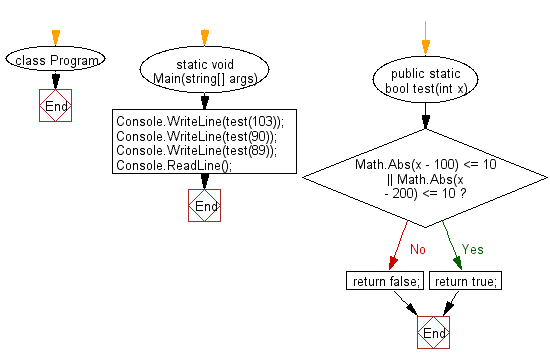﻿ C# - Check If an integer is between 10 and 100 or 200# C# Sharp Basic Algorithm Exercises: Check a given integer and return true if it is within 10 of 100 or 200

## C# Sharp Basic Algorithm: Exercise-4 with Solution

Write a C# Sharp program to check a given integer and return true if it is within 10 of 100 or 200.

Sample Solution:

C# Sharp Code:

``````using System;
namespace exercises
{
class Program
{
static void Main(string[] args)
{
Console.WriteLine(test(103));
Console.WriteLine(test(90));
Console.WriteLine(test(89));
}
public static bool test(int x)
{
if(Math.Abs(x - 100) <= 10 || Math.Abs(x - 200) <= 10)
return true;
return false;
}
}
}
```
```

Sample Output:

```True
True
False```

Flowchart:C# Sharp Code Editor:

Improve this sample solution and post your code through Disqus

What is the difficulty level of this exercise?

Test your Programming skills with w3resource's quiz.

﻿